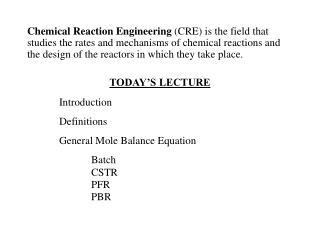# Chemical Reaction Engineering - PowerPoint PPT PresentationDownload PresentationChemical Reaction Engineering

Chemical Reaction Engineering
Download Presentation## Chemical Reaction Engineering

- - - - - - - - - - - - - - - - - - - - - - - - - - - E N D - - - - - - - - - - - - - - - - - - - - - - - - - - -
##### Presentation Transcript

1. Chemical Reaction Engineering (CRE) is the field that studies the rates and mechanisms of chemical reactions and the design of the reactors in which they take place. TODAY’S LECTURE Introduction Definitions General Mole Balance Equation Batch CSTR PFR PBR

2. Chemical Reaction Engineering Chemical reaction engineering is at the heart of virtually every chemical process. It separates the chemical engineer from other engineers. Industries that Draw Heavily on Chemical Reaction Engineering (CRE) are: CPI (Chemical Process Industries) Dow, DuPont, Amoco, Chevron

3. Materials on the Web and CDROM http://www.engin.umich.edu/~cre/

4. Developing Critical Thinking Skills Socratic Questioning is the Heart of Critical Thinking R. W. Paul’s Nine Types of Socratic Questions

5. Let’s Begin CRE Chemical Reaction Engineering (CRE) is the field that studies the rates and mechanisms of chemical reactions and the design of the reactors in which they take place.

6. Chemical Identity • A chemical species is said to have reacted when it has lost its chemical identity.

7. Chemical Identity • A chemical species is said to have reacted when it has lost its chemical identity. • The identity of a chemical species is determined by the kind, number, and configuration of that species’ atoms.

8. Chemical Identity • A chemical species is said to have reacted when it has lost its chemical identity. 1. Decomposition

9. Chemical Identity • A chemical species is said to have reacted when it has lost its chemical identity. 1. Decomposition 2. Combination

10. Chemical Identity • A chemical species is said to have reacted when it has lost its chemical identity. 1. Decomposition 2. Combination 3. Isomerization

11. Reaction Rate • The reaction rate is the rate at which a species looses its chemical identity per unit volume.

12. Reaction Rate • The reaction rate is the rate at which a species looses its chemical identity per unit volume. • The rate of a reaction (mol/dm3/s) can be expressed as either the rate of Disappearance: -rA or as the rate of Formation (Generation): rA

13. Reaction Rate Consider the isomerization AB rA = the rate of formation of species A per unit volume -rA = the rate of a disappearance of species A per unit volume rB = the rate of formation of species B per unit volume

14. Reaction Rate • EXAMPLE: AB If Species B is being formed at a rate of 0.2 moles per decimeter cubed per second, ie, rB = 0.2 mole/dm3/s

15. Reaction Rate • EXAMPLE: AB rB = 0.2 mole/dm3/s Then A is disappearing at the same rate: -rA= 0.2 mole/dm3/s

16. Reaction Rate • EXAMPLE: AB rB = 0.2 mole/dm3/s Then A is disappearing at the same rate: -rA= 0.2 mole/dm3/s The rate of formation (generation of A) is rA= -0.2 mole/dm3/s

17. Reaction Rate • For a catalytic reaction, we refer to -rA', which is the rate of disappearance of species A on a per mass of catalyst basis. (mol/gcat/s) NOTE: dCA/dt is not the rate of reaction

18. Reaction Rate Consider species j: • rj is the rate of formation of species j per unit volume [e.g. mol/dm3/s]

19. Reaction Rate • rj is the rate of formation of species j per unit volume [e.g. mol/dm3*s] • rj is a function of concentration, temperature, pressure, and the type of catalyst (if any)

20. Reaction Rate • rj is the rate of formation of species j per unit volume [e.g. mol/dm3/s] • rj is a function of concentration, temperature, pressure, and the type of catalyst (if any) • rj is independent of the type of reaction system (batch reactor, plug flow reactor, etc.)

21. Reaction Rate • rj is the rate of formation of species j per unit volume [e.g. mol/dm3/s] • rj is a function of concentration, temperature, pressure, and the type of catalyst (if any) • rj is independent of the type of reaction system (batch, plug flow, etc.) • rj is an algebraic equation, not a differential equation

22. General Mole Balance

23. General Mole Balance

24. Batch Reactor Mole Balance

25. CSTRMole Balance

26. Plug Flow Reactor

27. Plug Flow Reactor Mole Balance PFR: The integral form is: This is the volume necessary to reduce the entering molar flow rate (mol/s) from FA0 to the exit molar flow rate of FA.

28. Packed Bed Reactor Mole Balance PBR The integral form to find the catalyst weight is:

29. Reactor Mole Balance Summary

30. Fast Forward to the Future Thursday March 20th, 2008 Reactors with Heat Effects

31. Production of Propylene Glycol in an Adiabatic CSTR

32. What are the exit conversion X and exit temperature T? Solution Let the reaction be represented by

33. KEEPING UP

34. Separations Filtration Distillation Adsorption These topics do not build upon one another

35. Reaction Engineering Mole Balance Rate Laws Stoichiometry These topics build upon one another